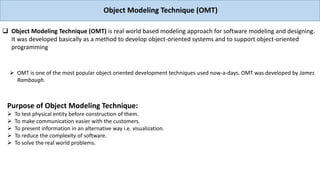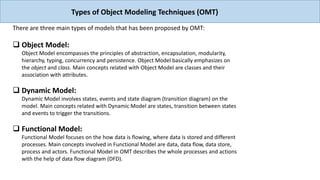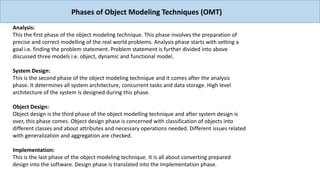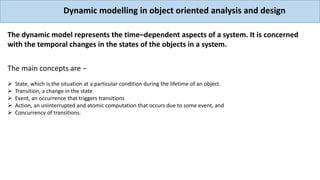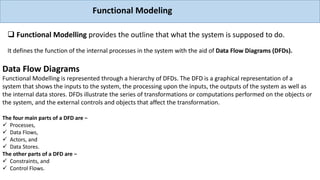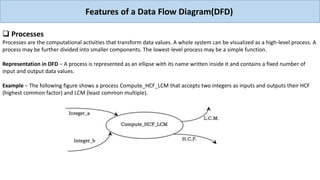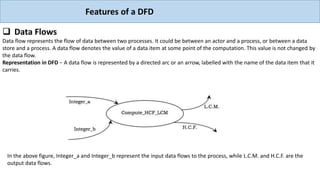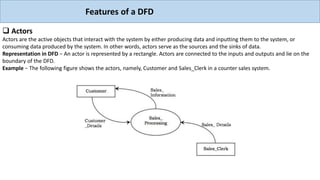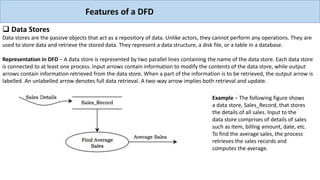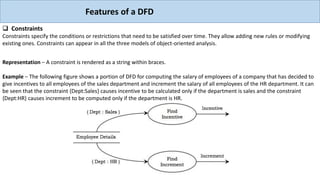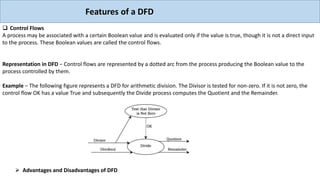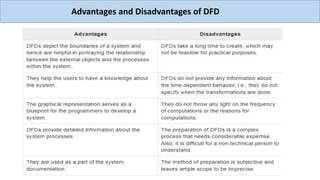1 of 12

1. Object Modeling Technique (OMT)  Object Modeling Technique (OMT) is real world based modeling approach for software modeling and designing. It was developed basically as a method to develop object-oriented systems and to support object-oriented programming  OMT is one of the most popular object oriented development techniques used now-a-days. OMT was developed by James Rambaugh. Purpose of Object Modeling Technique:  To test physical entity before construction of them.  To make communication easier with the customers.  To present information in an alternative way i.e. visualization.  To reduce the complexity of software.  To solve the real world problems.
2. There are three main types of models that has been proposed by OMT:  Object Model: Object Model encompasses the principles of abstraction, encapsulation, modularity, hierarchy, typing, concurrency and persistence. Object Model basically emphasizes on the object and class. Main concepts related with Object Model are classes and their association with attributes.  Dynamic Model: Dynamic Model involves states, events and state diagram (transition diagram) on the model. Main concepts related with Dynamic Model are states, transition between states and events to trigger the transitions.  Functional Model: Functional Model focuses on the how data is flowing, where data is stored and different processes. Main concepts involved in Functional Model are data, data flow, data store, process and actors. Functional Model in OMT describes the whole processes and actions with the help of data flow diagram (DFD). Types of Object Modeling Techniques (OMT)
3. Analysis: This the first phase of the object modeling technique. This phase involves the preparation of precise and correct modelling of the real world problems. Analysis phase starts with setting a goal i.e. finding the problem statement. Problem statement is further divided into above discussed three models i.e. object, dynamic and functional model. System Design: This is the second phase of the object modeling technique and it comes after the analysis phase. It determines all system architecture, concurrent tasks and data storage. High level architecture of the system is designed during this phase. Object Design: Object design is the third phase of the object modelling technique and after system design is over, this phase comes. Object design phase is concerned with classification of objects into different classes and about attributes and necessary operations needed. Different issues related with generalization and aggregation are checked. Implementation: This is the last phase of the object modeling technique. It is all about converting prepared design into the software. Design phase is translated into the Implementation phase. Phases of Object Modeling Techniques (OMT)
4. Dynamic modelling in object oriented analysis and design The dynamic model represents the time–dependent aspects of a system. It is concerned with the temporal changes in the states of the objects in a system. The main concepts are −  State, which is the situation at a particular condition during the lifetime of an object.  Transition, a change in the state  Event, an occurrence that triggers transitions  Action, an uninterrupted and atomic computation that occurs due to some event, and  Concurrency of transitions.
5. Functional Modeling  Functional Modelling provides the outline that what the system is supposed to do. It defines the function of the internal processes in the system with the aid of Data Flow Diagrams (DFDs). Data Flow Diagrams Functional Modelling is represented through a hierarchy of DFDs. The DFD is a graphical representation of a system that shows the inputs to the system, the processing upon the inputs, the outputs of the system as well as the internal data stores. DFDs illustrate the series of transformations or computations performed on the objects or the system, and the external controls and objects that affect the transformation. The four main parts of a DFD are −  Processes,  Data Flows,  Actors, and  Data Stores. The other parts of a DFD are −  Constraints, and  Control Flows.
6. Features of a Data Flow Diagram(DFD)  Processes Processes are the computational activities that transform data values. A whole system can be visualized as a high-level process. A process may be further divided into smaller components. The lowest-level process may be a simple function. Representation in DFD − A process is represented as an ellipse with its name written inside it and contains a fixed number of input and output data values. Example − The following figure shows a process Compute_HCF_LCM that accepts two integers as inputs and outputs their HCF (highest common factor) and LCM (least common multiple).
7. Features of a DFD  Data Flows Data flow represents the flow of data between two processes. It could be between an actor and a process, or between a data store and a process. A data flow denotes the value of a data item at some point of the computation. This value is not changed by the data flow. Representation in DFD − A data flow is represented by a directed arc or an arrow, labelled with the name of the data item that it carries. In the above figure, Integer_a and Integer_b represent the input data flows to the process, while L.C.M. and H.C.F. are the output data flows.
8. Features of a DFD  Actors Actors are the active objects that interact with the system by either producing data and inputting them to the system, or consuming data produced by the system. In other words, actors serve as the sources and the sinks of data. Representation in DFD − An actor is represented by a rectangle. Actors are connected to the inputs and outputs and lie on the boundary of the DFD. Example − The following figure shows the actors, namely, Customer and Sales_Clerk in a counter sales system.
9. Features of a DFD  Data Stores Data stores are the passive objects that act as a repository of data. Unlike actors, they cannot perform any operations. They are used to store data and retrieve the stored data. They represent a data structure, a disk file, or a table in a database. Representation in DFD − A data store is represented by two parallel lines containing the name of the data store. Each data store is connected to at least one process. Input arrows contain information to modify the contents of the data store, while output arrows contain information retrieved from the data store. When a part of the information is to be retrieved, the output arrow is labelled. An unlabelled arrow denotes full data retrieval. A two-way arrow implies both retrieval and update. Example − The following figure shows a data store, Sales_Record, that stores the details of all sales. Input to the data store comprises of details of sales such as item, billing amount, date, etc. To find the average sales, the process retrieves the sales records and computes the average.
10. Features of a DFD  Constraints Constraints specify the conditions or restrictions that need to be satisfied over time. They allow adding new rules or modifying existing ones. Constraints can appear in all the three models of object-oriented analysis. Representation − A constraint is rendered as a string within braces. Example − The following figure shows a portion of DFD for computing the salary of employees of a company that has decided to give incentives to all employees of the sales department and increment the salary of all employees of the HR department. It can be seen that the constraint {Dept:Sales} causes incentive to be calculated only if the department is sales and the constraint {Dept:HR} causes increment to be computed only if the department is HR.
11. Features of a DFD  Control Flows A process may be associated with a certain Boolean value and is evaluated only if the value is true, though it is not a direct input to the process. These Boolean values are called the control flows. Representation in DFD − Control flows are represented by a dotted arc from the process producing the Boolean value to the process controlled by them. Example − The following figure represents a DFD for arithmetic division. The Divisor is tested for non-zero. If it is not zero, the control flow OK has a value True and subsequently the Divide process computes the Quotient and the Remainder.  Advantages and Disadvantages of DFD